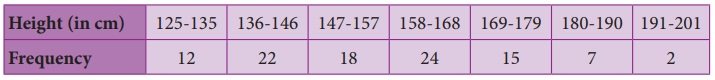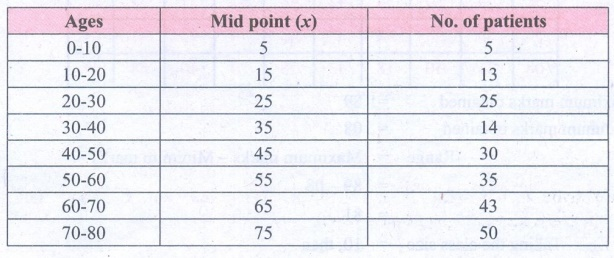Home | | Maths 8th Std | Exercise 6.2 (Graphical Representation of the Frequency Distribution for Grouped Data)

# Exercise 6.2 (Graphical Representation of the Frequency Distribution for Grouped Data)

8th Maths : Chapter 6 : Statistics : Graphical Representation of the Frequency Distribution for Grouped Data : Exercise 6.2 : Text Book Back Numerical problems, Exercises Questions with Answers, Solution

Exercise 6.2

1. Which of the following data can be represented in a histogram?

(i) The number of mountain climbers in the age group 20 to 60 in TamilNadu. [Answer: Yes]

(ii) Production of cycles in different years. [Answer: No]

(iii) The number of students in each class of a school. [Answer: No]

(iv) The number votes polled from 7 am to 6 pm in an election. [Answer: Yes]

(v) The wickets fallen from 1 over to 50th over in a one day cricket match. [Answer: Yes]

2. Fill in the blanks:

(i) The total area of the histogram is ________ to the total frequency of the given data. [Answer: proportional]

(ii) A graph that displays data that changes continuously over the periods of time is _____. [Answer: Histogram]

(iii) Histogram is a graphical representation of ___________ data. [Answer: grouped]

3. In a village, there are 570 people who have cell phones. An NGO survey their cell phone usage. Based on this survey a histogram is drawn. Answer the following questions.(i) How many people use the cell phone for less than 3 hours?

Solution: 330 people (110 + 220)

(ii) How many of them use the cell phone for more than 5 hours?

Solution: 150 of them (100 + 50)

(iii) Are people using cell phone for less than 1 hour?

4. Draw a histogram for the following data.Solution:

The given data is continuous frequency distribution taking class intervals on X axis and No. of students on Y−axis, the histogram is given below.5. Construct a histogram from the following distribution of total marks of 40 students in a class.Solution:

The given distribution is continuous taking marks on X axis and No. of students on Y−axis the histogram is constructed.6. The distribution of heights ( in cm ) of 100 people is given below. Construct a histogram and the frequency polygon imposed on it.Solution:

The given distribution is discontinuous.

Converting into continuous distribution we have

Lower boundary = lower limit – 1/2 (gap between adjacent class interval)

= 125 – 1/2 (1) = 124.5

Upper boundary = Upper limit + 1/2 (gap between the adjacent class interval)

= 135 + 1/2 = 135.5

The new frequency table is7. In a study of dental problem, the following data were obtained.Represent the above data by a frequency polygon.

Solution:

Finding the midpoints of the class interval we get.The points to be plotted are A (5,5), B (15, 13), C (25, 25), D (35, 14), E (45, 30), F(55,35) G (65, 43.), H (75, 50) to obtain the frequency polygon ZABCDEFGHI.

Where I imagined class between 80 and 90.8. The marks obtained by 50 students in Mathematics are given below (i) Make a frequency distribution table taking a class size of 10 marks (ii) Draw a histogram and a frequency polygon.Solution:

Maximum marks obtained = 89

Minimum marks obtained = 08

Range =  Maximum marks − Minimum marks

= 89 – 08

= 81

Taking the class size = 10, then

Number of possible intervals = Range / Class size

= 81/10 = 8.1 = 9Now we have the continuous frequency table.We will draw the histogram taking class interval in x axis and frequency in y axis as follows.Objective Type Questions

9. Data is a collection of ________

(A) numbers

(B) words

(C) measurements

(D) all the three

10. The number of times an observation occurs in the given data is called ________

(A) tally marks

(B) data

(C) frequency

(D) none of these

11. The difference between the largest value and the smallest value of the given data is ________

(A) range

(B) frequency

(C) variable

(D) none of these

12. The data that can take values between a certain range is called________

(A) ungrouped

(B) grouped

(C) frequency

(D) none of these

13. Inclusive series is a ________series.

(A) continuous

(B) discontinuous

(C) both

(D) none of these

14. In a class interval the upper limit of one class is the lower limit of the other class. This is ________series.

(A) Inclusive

(B) exclusive

(C) ungrouped

(D) none of these

15. The graphical representation of ungrouped data is ________

(A) histogram

(B) frequency polygon

(C)pie chart

(D) all the three

16. Histogram is a graph of a ________ frequency distribution.

(A) continuous

(B) discontinuous

(C) discrete

(D) none of these

17. A ________ is a line graph for the graphical representation of the continuous frequency distribution.

(A) frequency polygon

(B) histogram

(C) pie chart

(D) bar graph

18. The graphical representation of grouped data is ________

(A) bar graph

(B) pictograph

(C) pie chart

(D) histogram

Exercise 6.2

1. (i) Yes (ii) No (iii) No (iv) Yes (v) Yes

2. (i) Proportional (ii) Histogram(iii) Grouped

3. (i) 330 (ii) 150 (iii) No

9. (d) all the three

10. (c) Frequency

11. (a) range

12. (b) grouped

13. (b) discontinuous

14. (b) Exclusive

15. (c) pie chart

16. (a) continuous

17. (a) frequency polygon

18. (d) histogram

Tags : Questions with Answers, Solution | Statistics | Chapter 6 | 8th Maths , 8th Maths : Chapter 6 : Statistics
Study Material, Lecturing Notes, Assignment, Reference, Wiki description explanation, brief detail
8th Maths : Chapter 6 : Statistics : Exercise 6.2 (Graphical Representation of the Frequency Distribution for Grouped Data) | Questions with Answers, Solution | Statistics | Chapter 6 | 8th Maths